# 5 視覺化：ggplot2

(投影片 / 影片)
Some of the material is based on Garrett Grolemund’s introduction to ggplot2

## 5.2 今天用到的資料: diamonds

• diamondsggplot2 套件的內建資料。這筆資料記錄著 5 萬多筆鑽石的售價以及各種資訊。可使用 ?diamonds 閱讀此資料各變項的說明。
carat cut color clarity depth table price x y z
0.23 Ideal E SI2 61.5 55 326 3.95 3.98 2.43
0.21 Premium E SI1 59.8 61 326 3.89 3.84 2.31
0.23 Good E VS1 56.9 65 327 4.05 4.07 2.31
0.29 Premium I VS2 62.4 58 334 4.20 4.23 2.63
0.31 Good J SI2 63.3 58 335 4.34 4.35 2.75
0.24 Very Good J VVS2 62.8 57 336 3.94 3.96 2.48

library(dplyr)
library(ggplot2)

diam <- diamonds %>% sample_n(size = 1500)

## 5.3 Template 1

• 最基本的 ggplot 模板：

ggplot(data = <DATA>) +
<GEOM_FUNCTION>(mapping = aes(<MAPPINGS>))
• 使用模板繪製散布圖：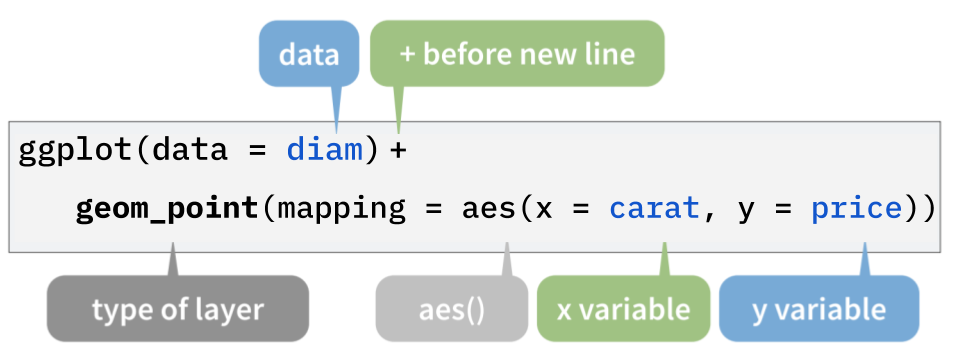Figure 5.1: ggplot() 的結構

### 5.3.1 散布圖 (Scatter plot)

• 使用下方程式碼，可繪製出鑽石的重量 (克拉)價錢 (美元) 的關係 (散布圖)

library(ggplot2)

ggplot(data = diam) +
geom_point(mapping = aes(x = carat, y = price))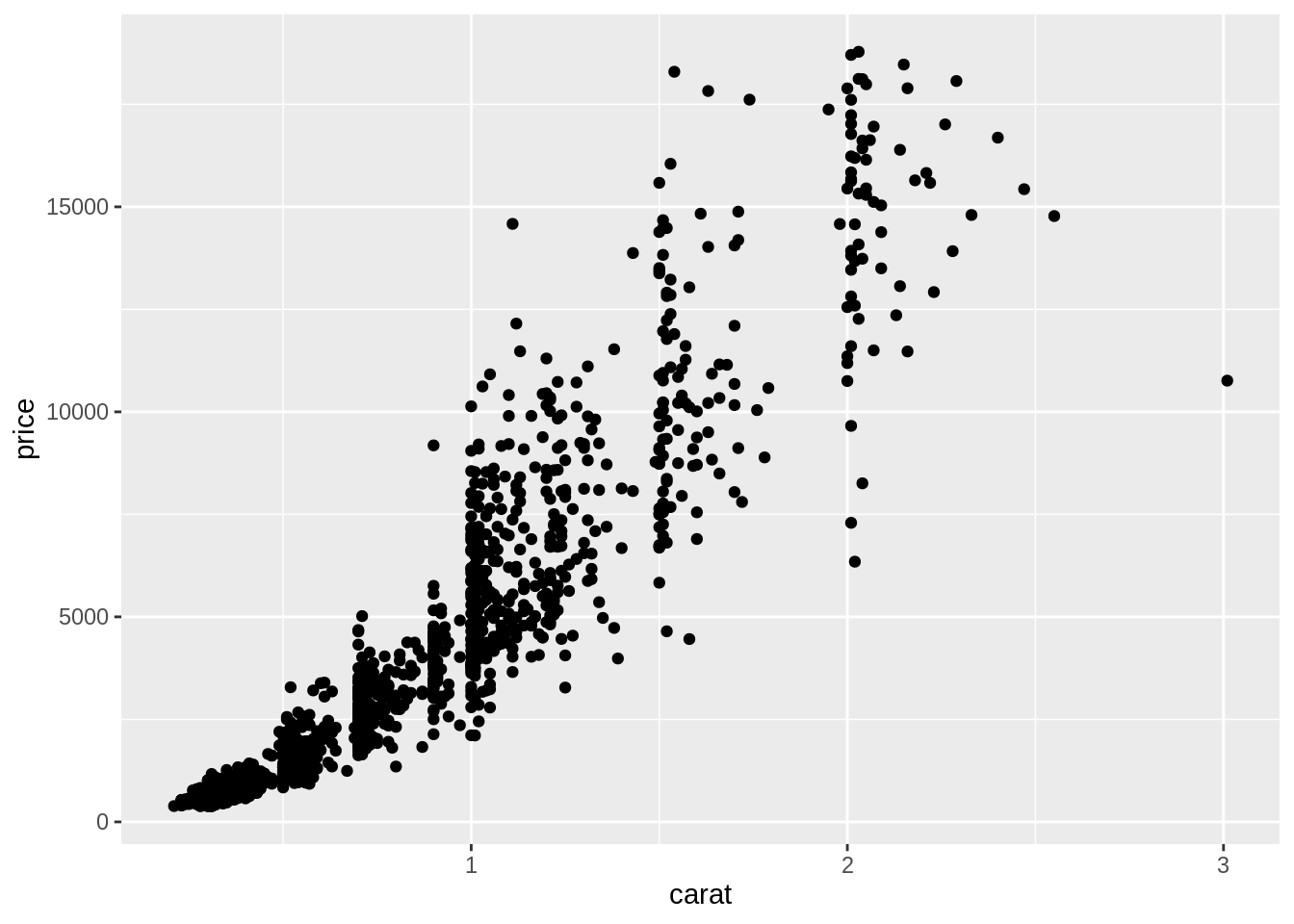## 5.4 圖層

• 在 ggplot 的概念中，圖片是由一層層圖層堆疊起來的：
1. 第一層 (ggplot()) 是底圖 (初始化繪圖函數)。在這層定義的內容 (e.g. data) 可被之後的圖層使用。

ggplot(data = diam)   # 底層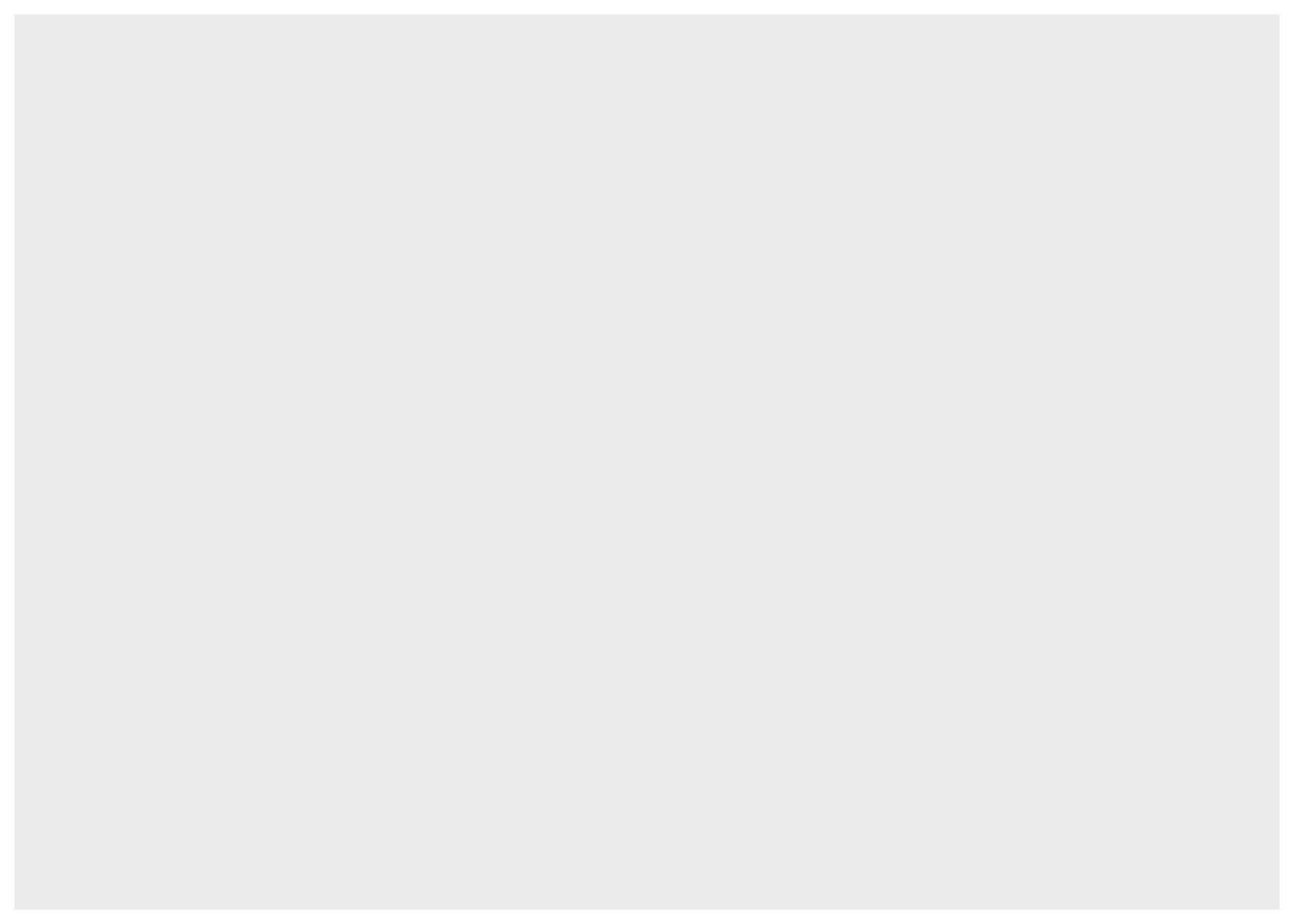2. 第二層 (geom_point()) 畫在底圖之上。圖層之間以 + 連結起來,要將第一與第二層連起來，得在繪製第一層圖層的程式碼之後加上一個 +

ggplot(data = diam) +                                # 底層
geom_point(mapping = aes(x = carat, y = price))    # 第二層3. 如果想增加其它圖層，只要再繼續使用 +:

ggplot(data = diam) +                                # 底層
geom_point(mapping = aes(x = carat, y = price)) +  # 第二層
geom_smooth(mapping = aes(x = carat, y = price))   # 第三層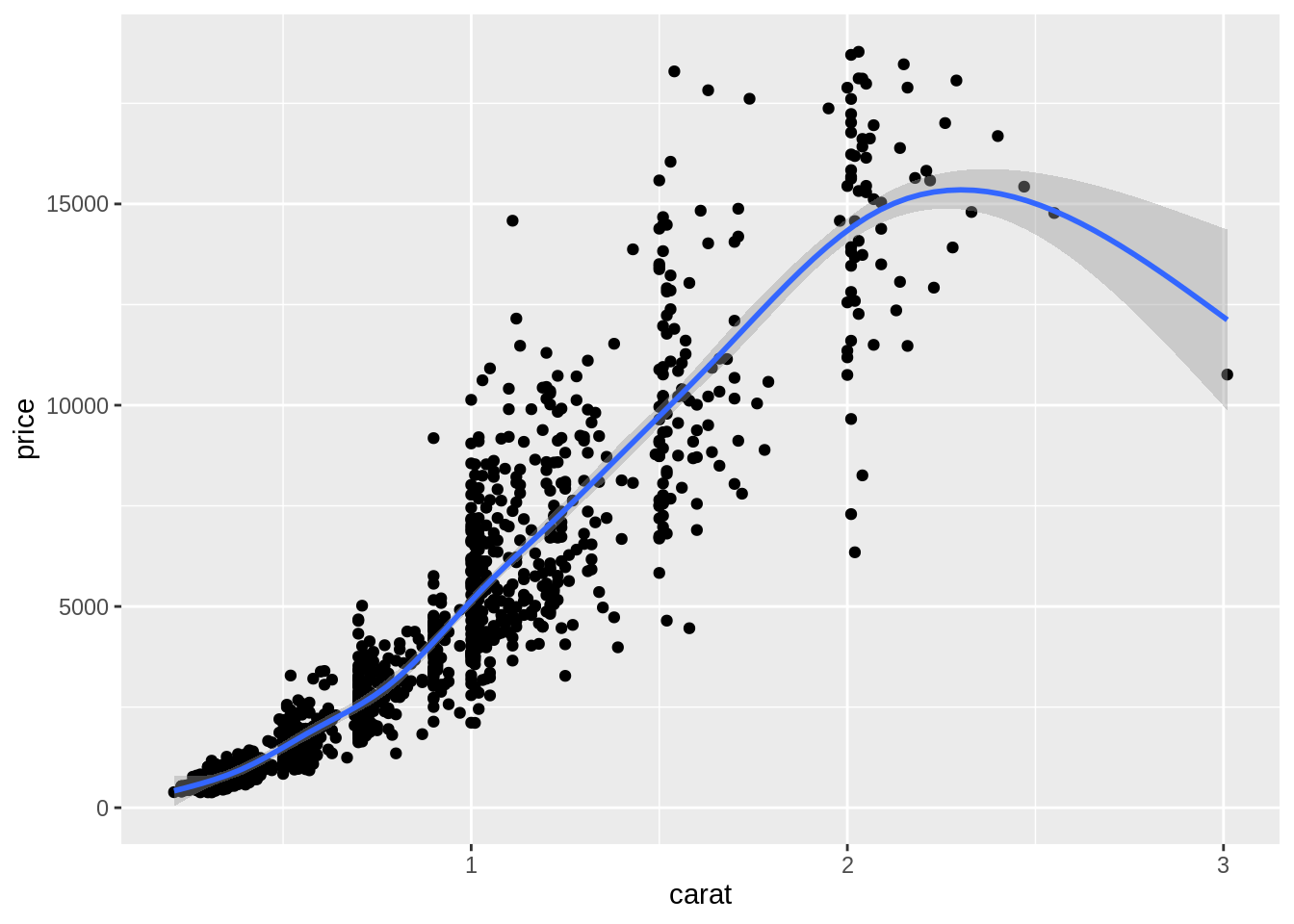## 5.5 Mapping: 將資料對應至視覺屬性

• 要能繪製統計圖，必須先將抽象的資料 (i.e. data frame) 對應至實際可見的視覺屬性上 (e.g. 位置、形狀、大小、顏色、透明度等) 。不同的統計圖，所需的「資料與視覺屬性間的對應關係」就不同。

• 要繪製一個散布圖，我們必須將 data frame 中的一個變項對應至 x 軸、另一個變項對應至 y 軸，以將 data frame 中的每筆觀察值 (抽象資料) 轉換成圖上的一個個點 (視覺屬性)

• geom_*() 中的參數 mapping，即在定義「data frame 中的資料與統計圖上之視覺屬性的關係」：

ggplot(data = diam) +
geom_point(mapping = aes(x = carat, y = price, color = clarity))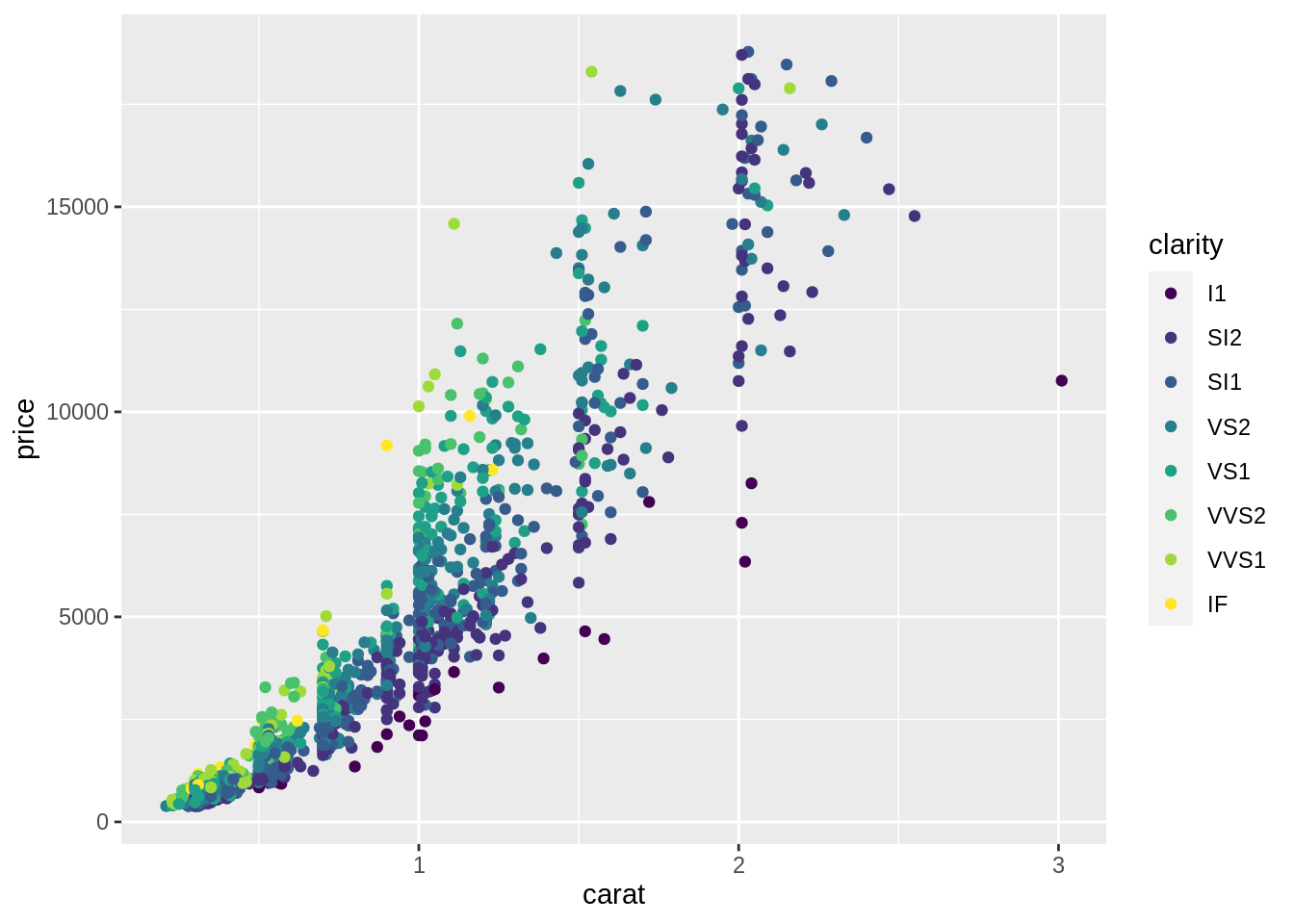• aes() 裡面定義資料與視覺屬性間的關係
• x = carat: 將 diam 中的變項 carat 對應至散布圖的 x 軸
• y = price: 將 diam 中的變項 price 對應至散布圖的 y 軸
• xy 會共同決定一個點的位置
• color = clarity: 將 diam 中的變項 clarity 對應至散布圖上點的顏色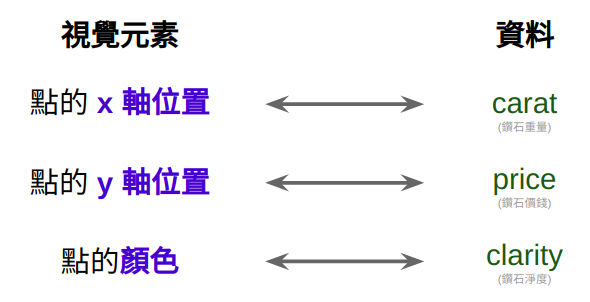Figure 5.2: Aesthetic Mappings

## 5.6 長條圖 (Bar chart)

• 概念上，繪製長條圖與繪製散布圖是很不一樣的：

• 散布圖上的視覺屬性可以直接對應到 data frame 裡的資料
• 長條圖的視覺屬性 (bar 的長度) 無法直接對應到 data frame 裡的資料。它對應到的是由 data frame 裡的資料經過彙整的結果:
• x 軸上是某變項裡的各個類別組成的
• y 軸代表各個類別出現的次數
• geom_bar() 是用來繪製長條圖的函數。在定義 mapping 時，只需將 data frame 的某個變項 (通常為類別變項) 對應至 xgeom_bar() 即會自動依據此變項計算出各類別的次數。換言之，在生成的長條圖中，y 軸 (count) 並非 data frame 的變項，而是 geom_bar() 幫你計算出來的東西，因此在定義 mapping 時，不需定義變項與 y 軸間的 mapping。

ggplot(data = diam) +
geom_bar(mapping = aes(x = cut))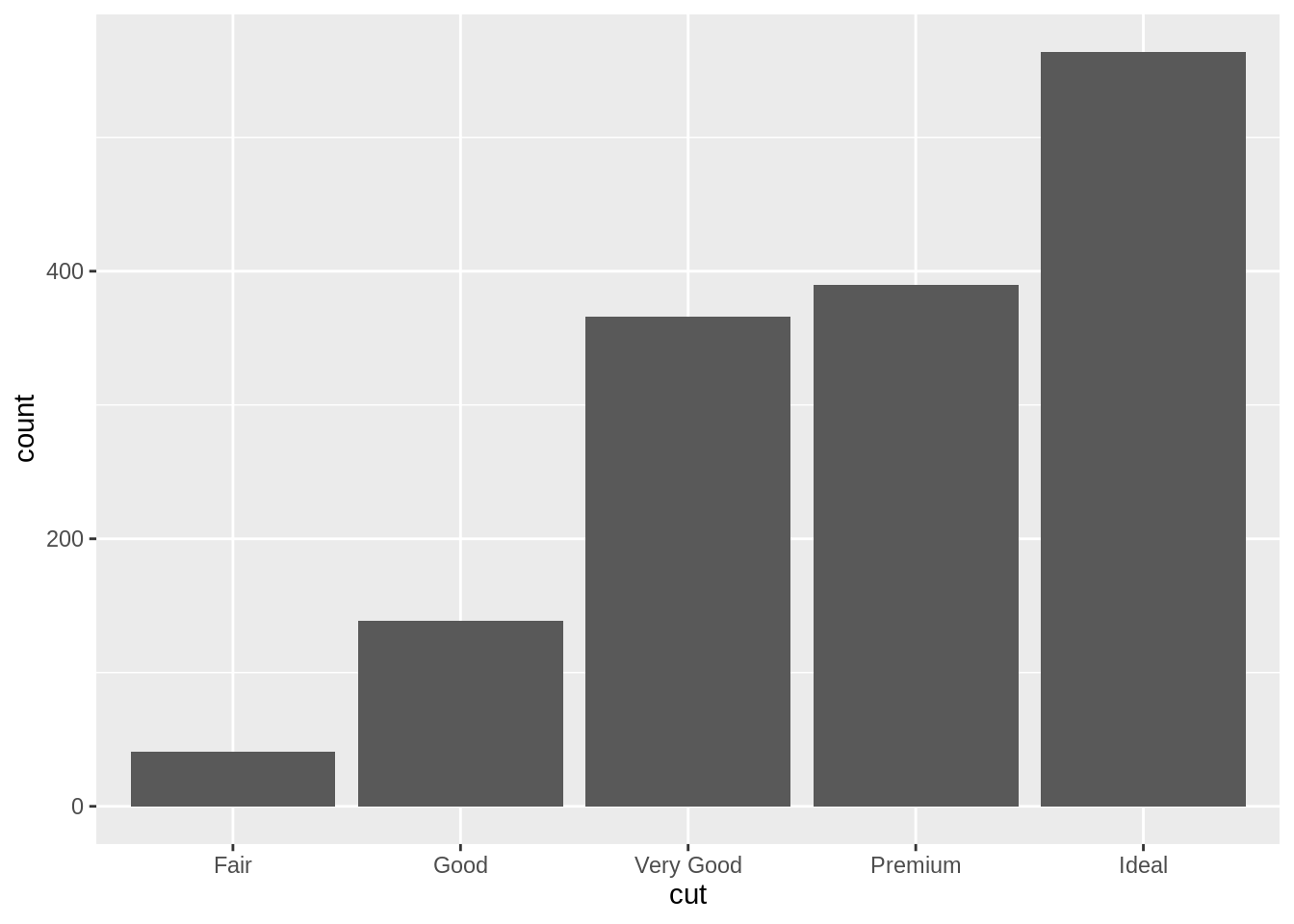• geom_bar() 在繪製長條圖之前，為符合繪圖需求而將傳入的 data frame 進行的運算，稱為 Statistical Transformation: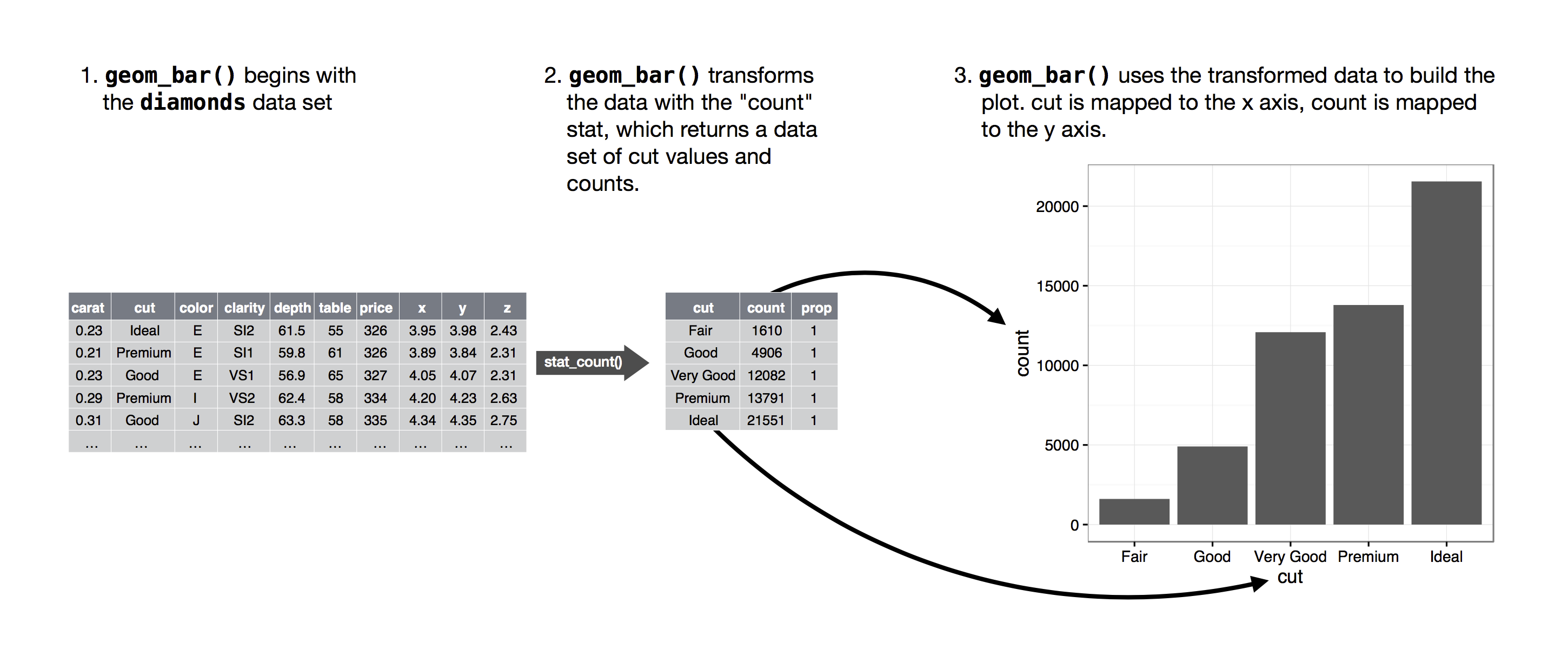Figure 5.3: geom_bar()’s default statistical transformation13

## 5.7 Statistical Transformations

• 有時候，我們只能拿到已整理好的資料，換言之，我們無法從 x 裡面去計算出裡面各類別的次數 (count)，例如 sum_data 已儲存著 cut 當中各類別的次數 (count)：

sum_data <- diam %>%
group_by(cut) %>%
summarise(count = n())

sum_data
#> # A tibble: 5 x 2
#>   cut       count
#>   <ord>     <int>
#> 1 Fair         41
#> 2 Good        139
#> 3 Very Good   366
#> 5 Ideal       564
• 如果想使用 sum_data 之中的變項直接去畫出長條圖，就需要覆寫 「geom_bar() 自動從 x 計算出次數」的預設行為。這個行為可由 geom_bar()stat 參數進行設定。

• geom_bar()stat 預設值是 "count"，讓 geom_bar() 可以從「對應至 x 的變項」計算出此變項裡各類別的次數。

• 若不想自動進行這種計算，而想直接使用 data frame 本身的變項，則可以將 stat 設為 "identity"，此時便可以在 mapping直接將 data frame 的變項對應至長條圖的 x 軸 與 y 軸：

# stat = "identity"
ggplot(data = sum_data) +
geom_bar(mapping = aes(x = cut, y = count),
stat = "identity") +
labs(title = 'stat = "identity"')

# stat = "count" (geom_bar 預設)
ggplot(data = diam) +
geom_bar(mapping = aes(x = cut),
stat = "count") +
labs(title = 'stat = "count" (default)')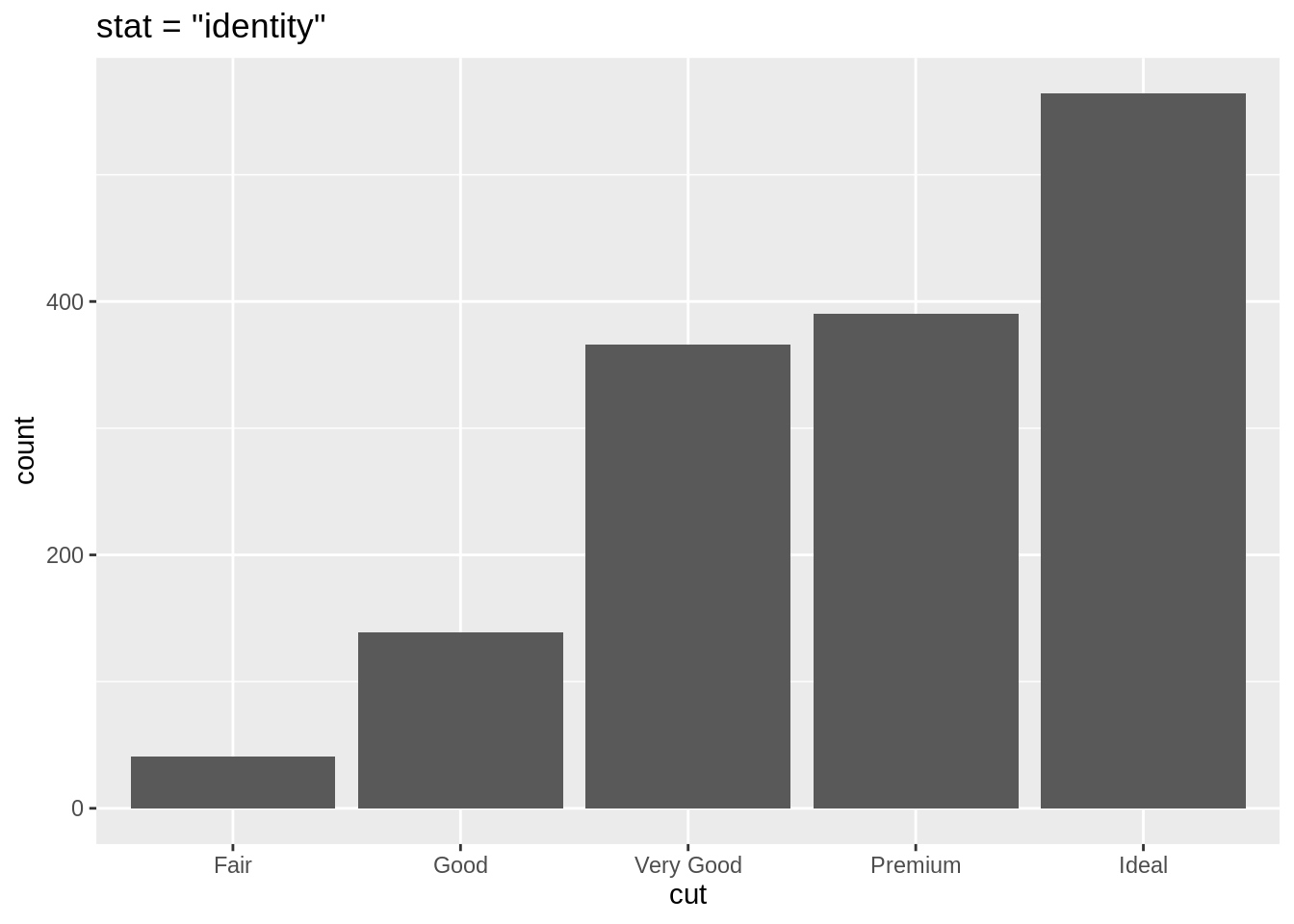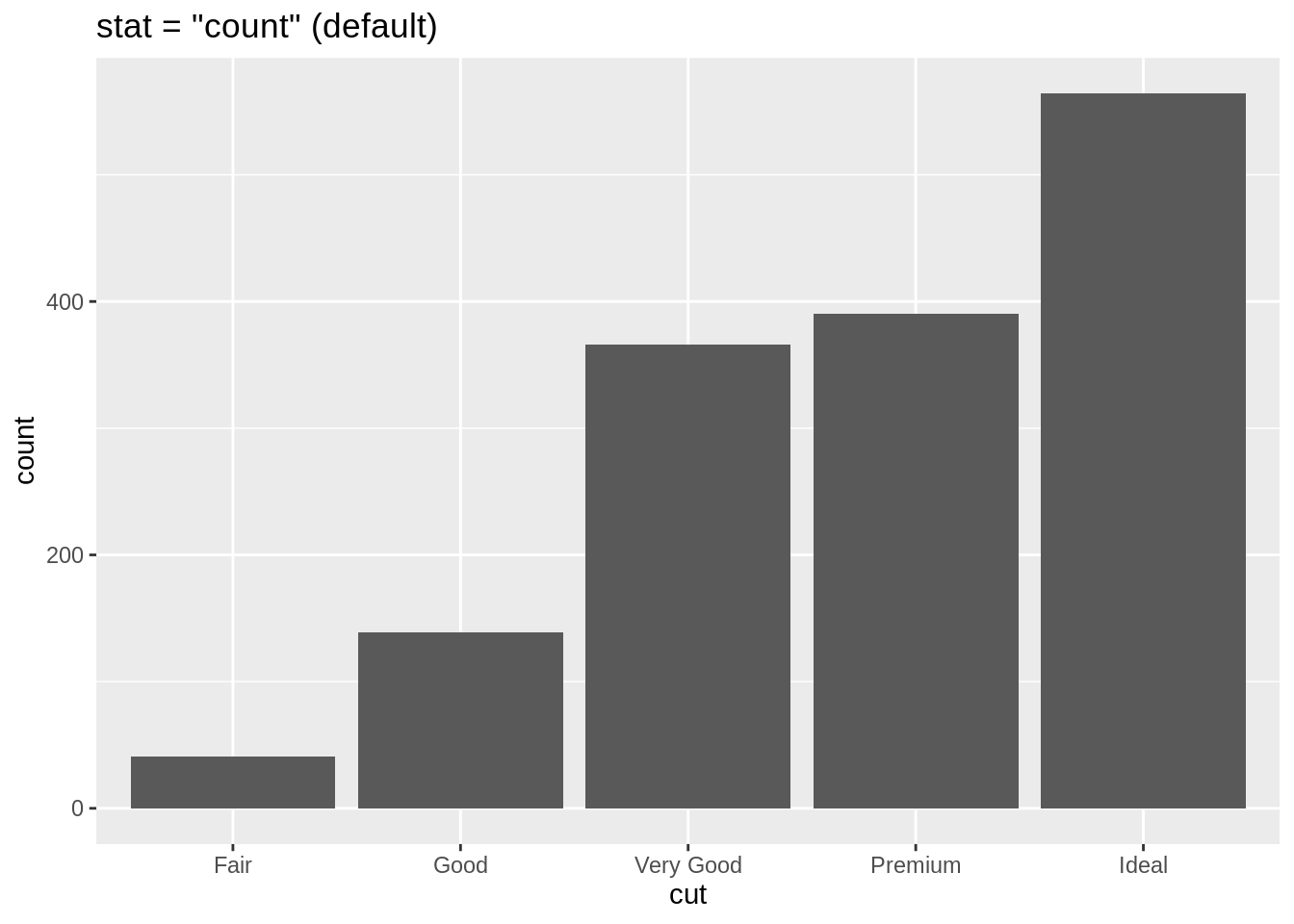### 5.7.1geom_*() 與 stat 預設

• 所有的 geom_*() 函數都會有一個 stat 的預設值：
• geom_point() 預設 stat = "identity"，所以給定 xy 兩個 mapping，便會將 data frame 中的變項對應至圖上的 x 軸與 y 軸。
• geom_bar() 預設 stat = "count"，會從對應至 x 的變項中計算出該變項各個類別的次數，並將此次數繪於圖上的 y 軸

• 透過提供第二個 mapping, fill, geom_bar 能夠將每個長條再依據第二個類別變項進行細分。
ggplot(data = diam) +
geom_bar(aes(x = cut, fill = clarity))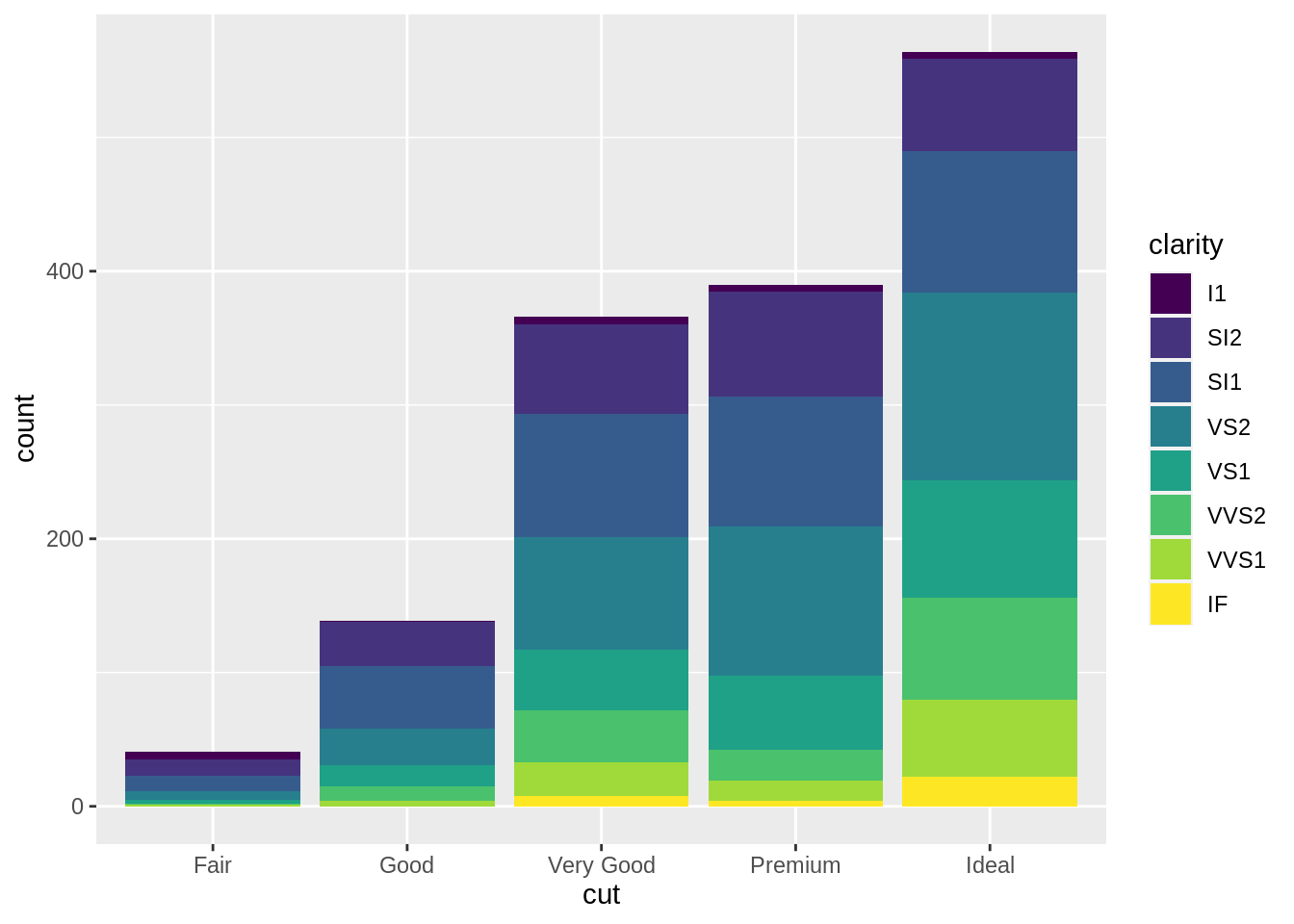# position: stack (default)
ggplot(data = diam) +
geom_bar(aes(x = cut, fill = clarity),
position = "stack")

# position: fill
ggplot(data = diam) +
geom_bar(aes(x = cut, fill = clarity),
position = "fill")

# postion: dodge
ggplot(data = diam) +
geom_bar(aes(x = cut, fill = clarity),
position = "dodge")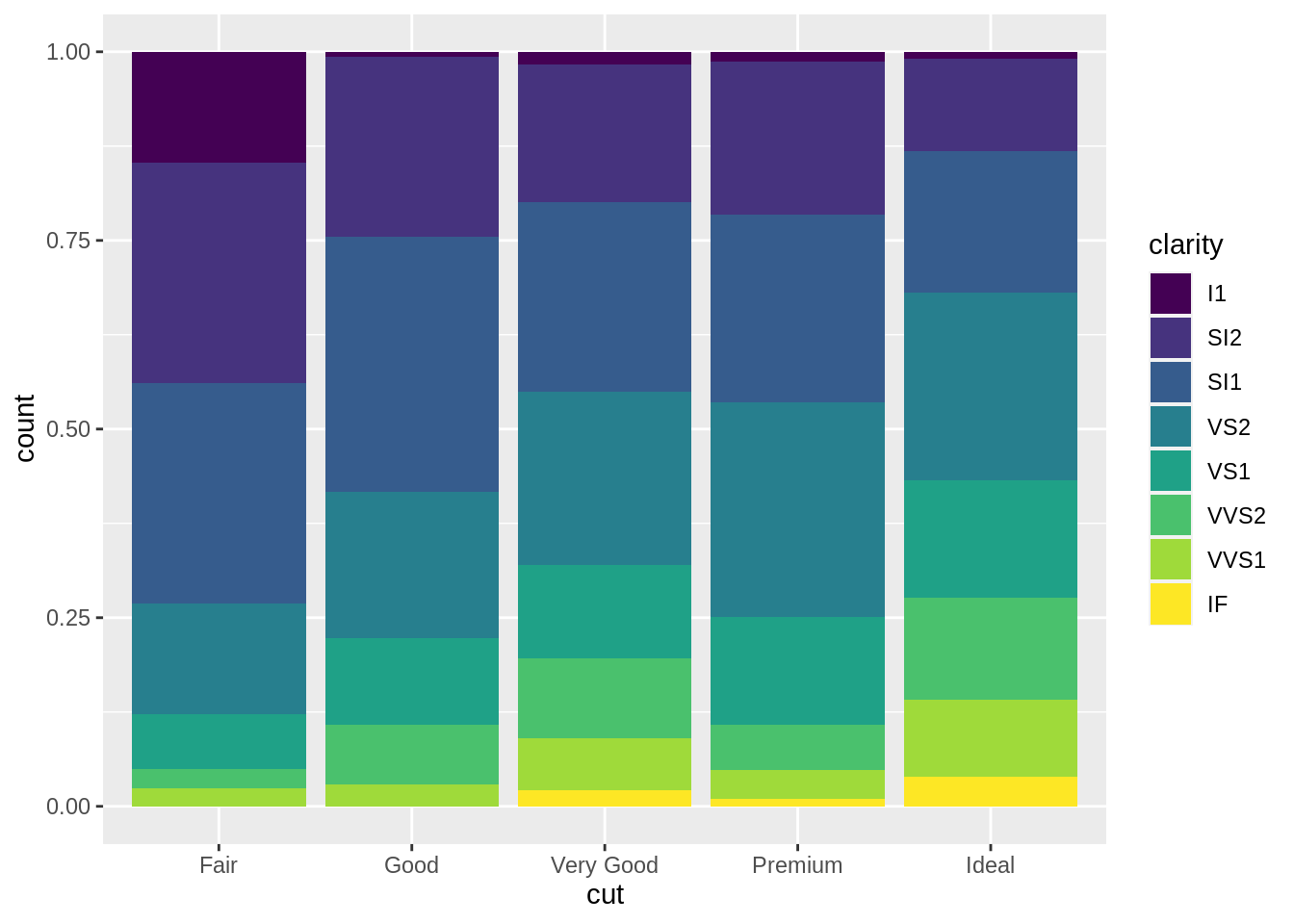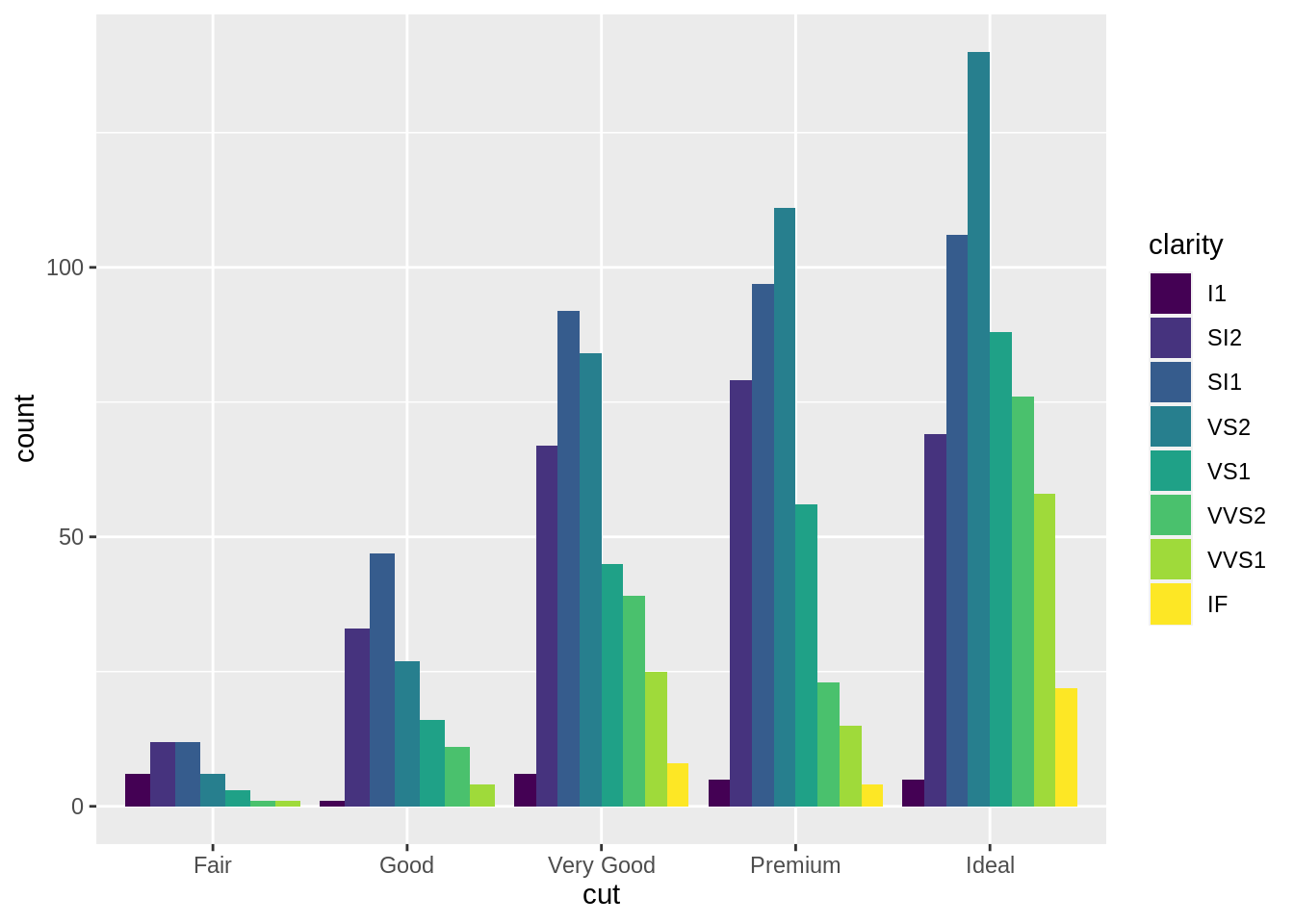## 5.9 Template 2

ggplot(data = <DATA>) +
<GEOM_FUNCTION>(
mapping = aes(<MAPPINGS>),
stat = <STAT>,
position = <POSITION>
)

## 5.10 Facet

• 1 個類別變項

ggplot(data = diam) +
geom_point(aes(x = carat, y = price)) +
facet_wrap(vars(cut))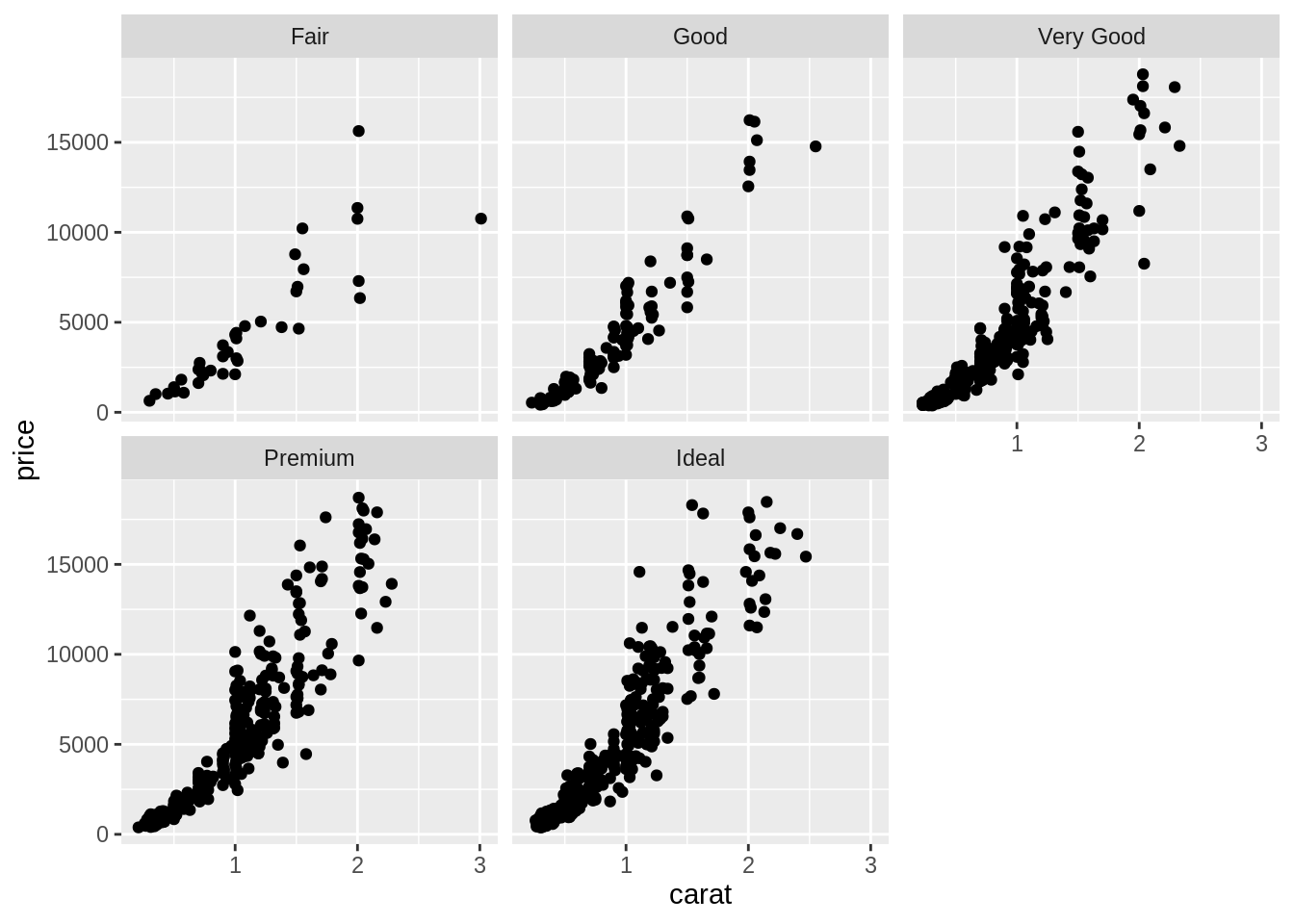• 2 個類別變項

ggplot(data = diam) +
geom_point(mapping = aes(x = carat,
y = price)) +
facet_grid(rows = vars(cut),
cols = vars(clarity))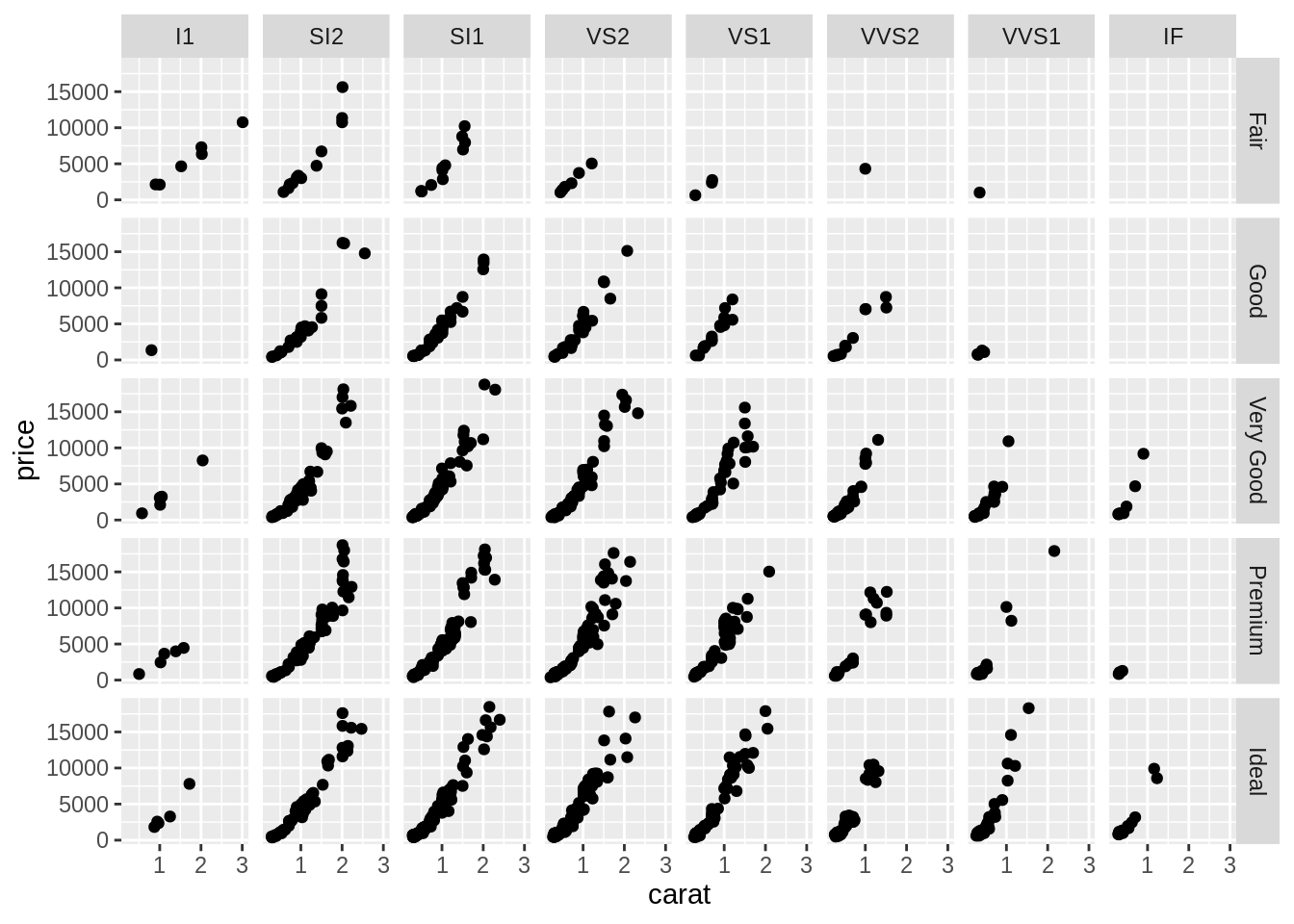## 5.11 Template 3

ggplot(data = <DATA>) +
<GEOM_FUNCTION>(
mapping = aes(<MAPPINGS>),
stat = <STAT>,
position = <POSITION>
) +
<FACET_FUNCTION>

## 5.12 Geoms

ggplot(mpg) +
geom_point(aes(class, hwy))
#ggsave('mpg_class_hwy_point.png', width = 14, height = 12, units = 'cm')

ggplot(mpg) +
geom_boxplot(aes(class, hwy))
#ggsave('mpg_class_hwy_boxplot.png', width = 14, height = 12, units = 'cm')

ggplot(mpg) +
geom_point(aes(class, hwy, color = drv))
#ggsave('mpg_class_hwy_color.png', width = 16, height = 12, units = 'cm')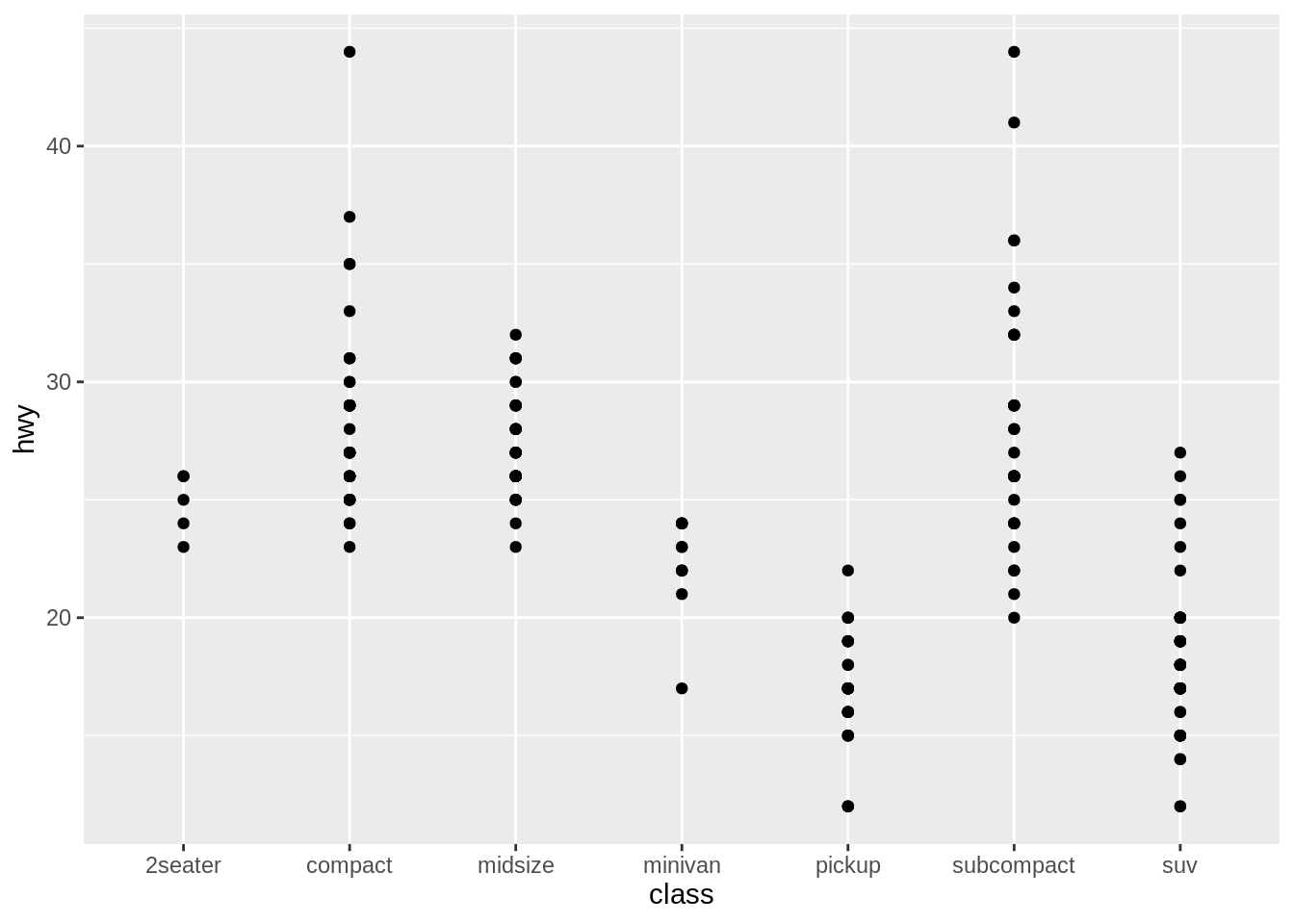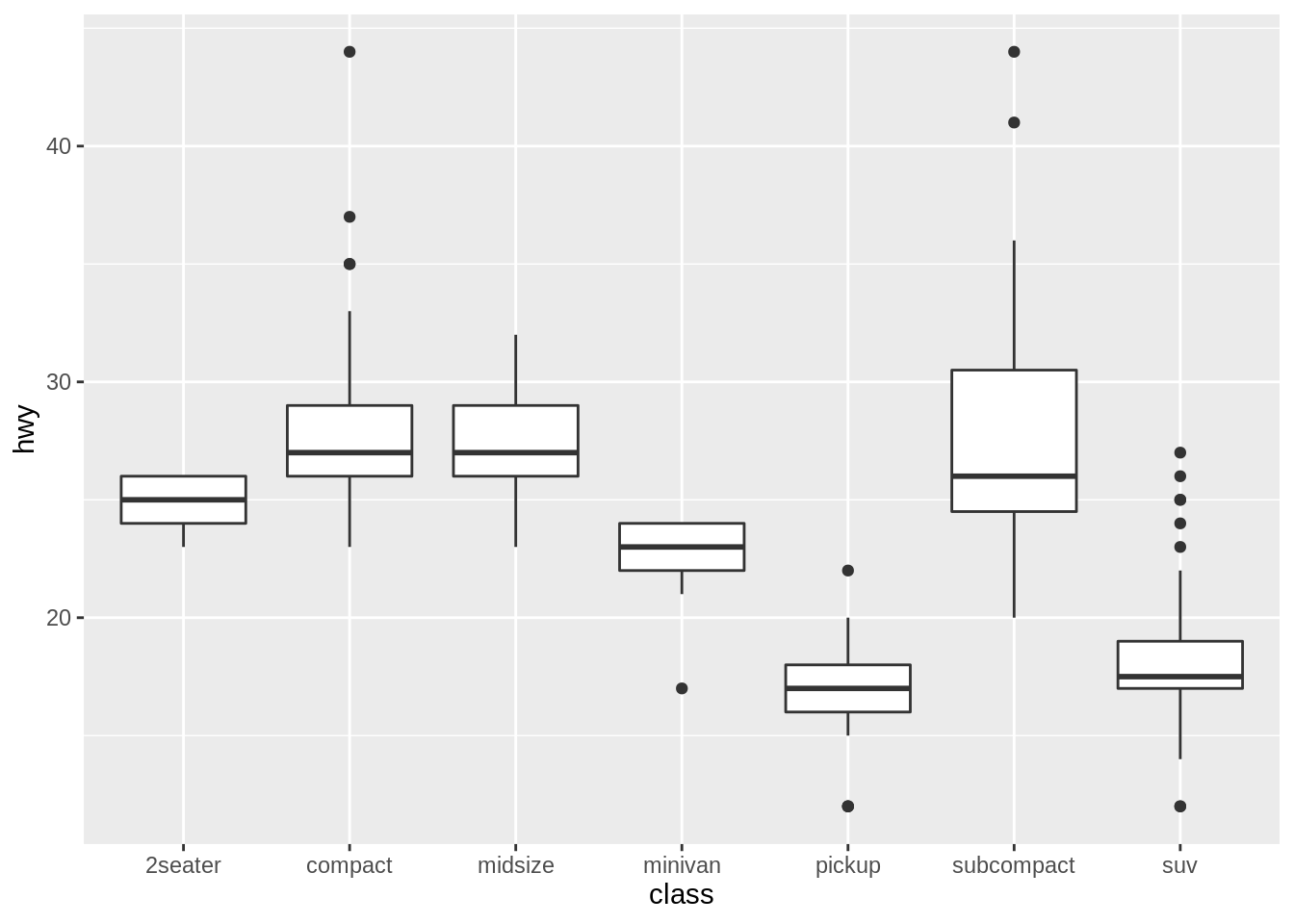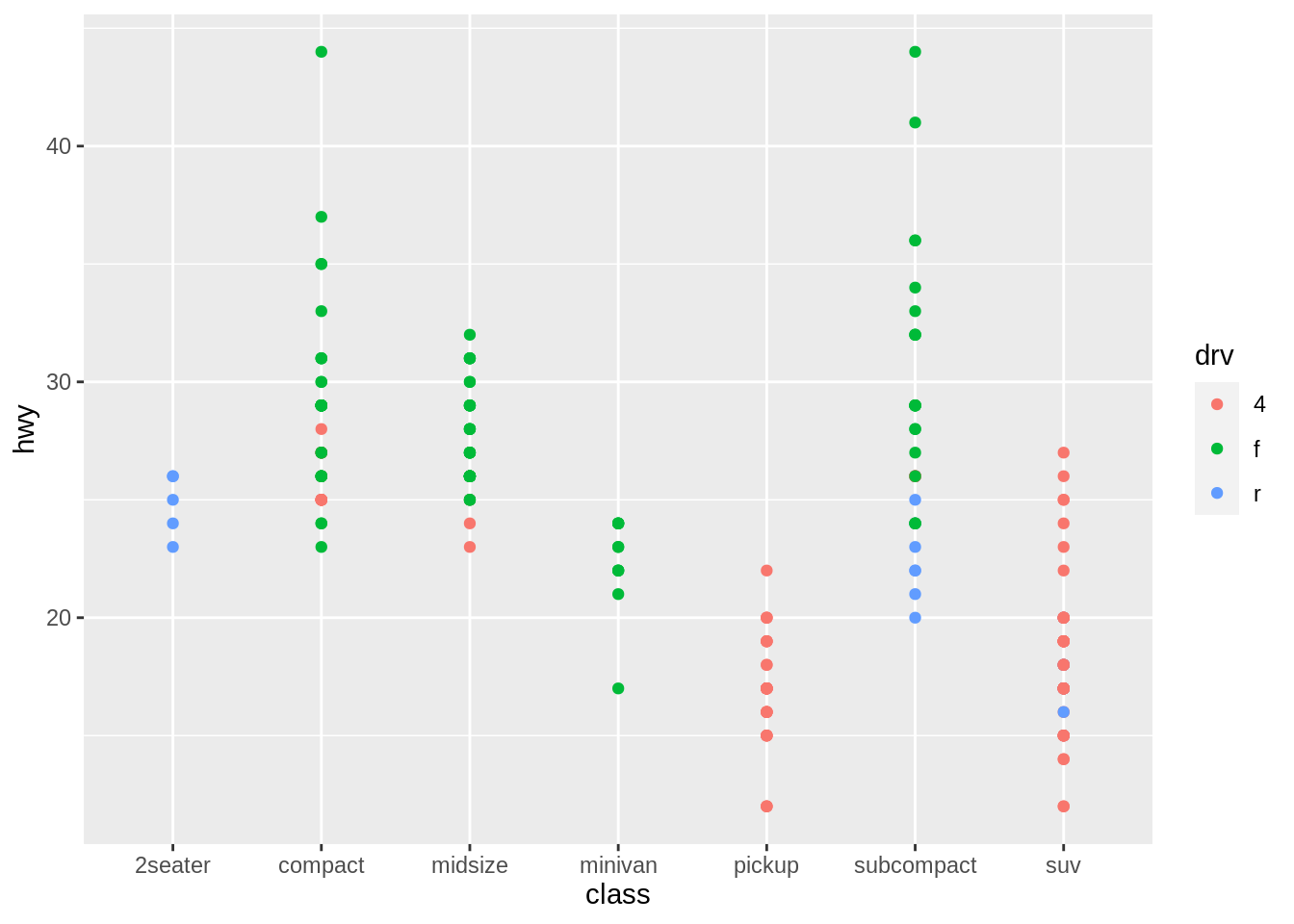## 參考資源 (務必閱讀)

1. Wickham, H., & Grolemund, G. (2017). R for Data Science: Data visualisation